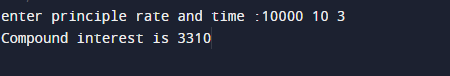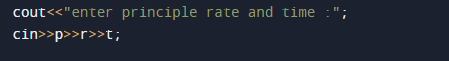# C++ Program to Calculate Compound Interest

In this tutorial you will learn about the C++ Program to Calculate Compound Interest and its application with practical example.

In this tutorial, we will learn to create a c++ program that will calculate compound interest using c++ programming.

## Prerequisites

Before starting with this tutorial we assume that you are best aware of the following C programming topics:

• Arithmetic operators.
•  Basic input/output.
• Looping statements.
• Operator precedence.
• Data types.

## What Is Compound Interest?

Compound Interest is a interest that obtain by adding the interest of each year to the principal sum and then calculating the interest on the updated amount.

CI is interest earned from the original principal +  accumulated interest.

## Formula for Compound Interest is.Here,
P is principle amount.
R is the rate in Percent %.
T is time in year.

## C++ Program to Calculate Compound Interest.

In this program we will find Compound Interest ,We would first declared and initialized the required variables. Next, we would prompt user to input principle,rate and time (p, r, t) and  applying Compound Interest formula to calculate Compound Interest. Now let’s have look at the code .

## Output## Here the Logic behind to calculate compound interest.

Here is the  logic to find compound interest.

• First of all we take 3 values of  principle,time and  rate.• We store the given inputted values in variable p,rand t respectively.
• Now passing all three values to know formula of CI which is.First of all the values of  p,r and t is given by user at compile time and with the help of power function we will calculate the ci of given years.Finally, we print the resultant value of ci.In this tutorial we have learn about the C++ Program to Calculate Compound Interest and its application with practical example. I hope you will like this tutorial.